# How to Calculate the Median in SPSS

This quick tutorial will show how to calculate the median of a set of data in SPSS.

### Quick Steps

1. Click Analyze -> Descriptive Statistics -> Frequencies
2. Move the variable for which you wish to calculate the median into the right-hand column
3. Click the Statistics button, select Median under Central Tendency, and then press Continue
4. Click OK to perform the calculation

## The Data

This is the data set with which we’re going to be working.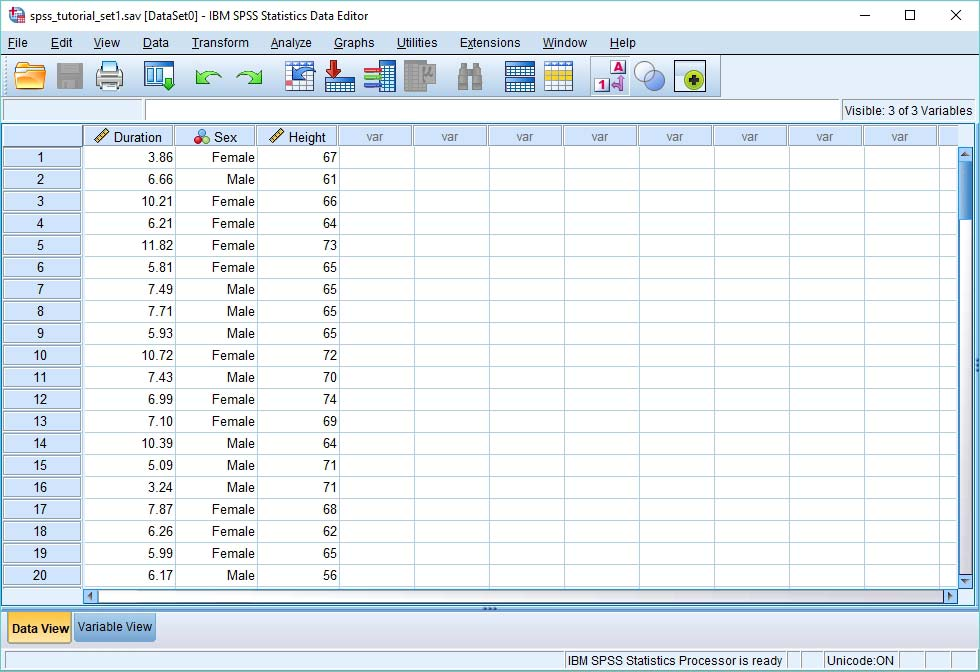So we’ve got three variables here: (a) duration – which is the duration in seconds it takes to complete a certain task; (b) sex – male or female; and (c) height – in inches.

You want to find out the median of the duration variable. In other words, you want to know the duration in seconds that lies exactly at the midpoint of the distribution of all durations.

## Calculate the Median

There are a number of different ways of calculating the median in SPSS. This is probably the easiest.

Click Analyze -> Descriptive Statistics -> Frequencies.

This will bring up the Frequencies dialog box.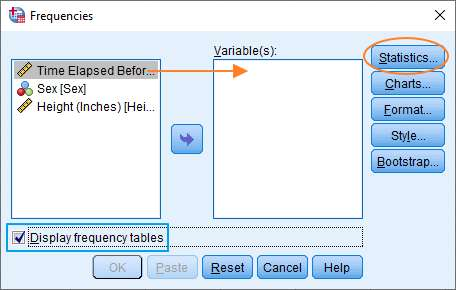You need to get the variable for which you wish to calculate the median into the Variable(s) box on the right. You can do this by dragging and dropping, or by selecting the variable on the left, and then clicking the arrow in the middle.

Once you’ve set this up, hit the Statistics button to bring up the Statistics dialog box.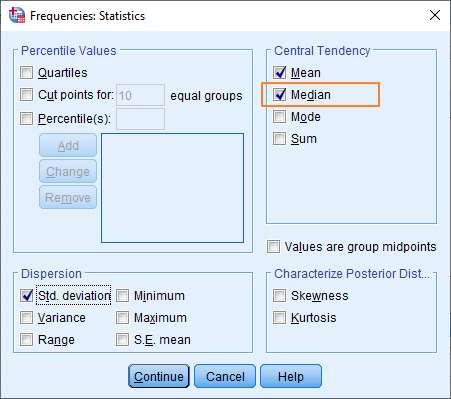Here you just want to tick the Median option under Central Tendency on the right.

We’ve also selected Mean and Standard Deviation, just because these are standard measures of central tendency and dispersion (respectively).

When you’re done, click Continue. You should now be looking at something like this.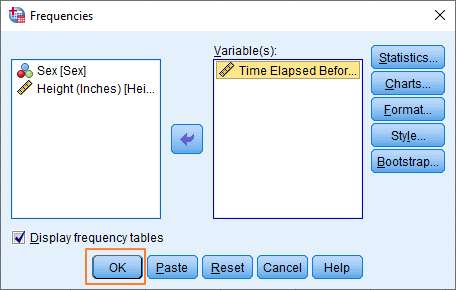It’s probably worth noting that we’ve also selected Display frequency tables at the bottom on the left. This isn’t necessary, but the option will provide useful additional information.

You’re now set up to calculate the median.

Just hit the OK button.

## The Result

The result appears in SPSS’s output viewer.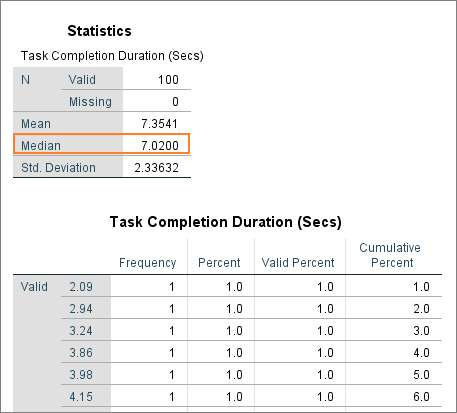As you can see, this is very easy to interpret.

For our example, the median value is 7.02. (The mean is 7.3541, and the standard deviation is 2.33632).

***************

That’s all for this quick tutorial. You should now know how to calculate the median in SPSS.